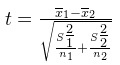# T-Test Formula

The t-test is any statistical hypothesis test in which the test statistic follows a Student’s t-distribution under the null hypothesis. It can be used to determine if two sets of data are significantly different from each other, and is most commonly applied when the test statistic would follow a normal distribution if the value of a scaling term in the test statistic were known.

T-test uses means and standard deviations of two samples to make a comparison. The formula for T-test is given below:

$\large t=\frac{\overline{x}_{1}-\overline{x}_{2}}{\sqrt{\frac{S_{1}^{2}}{n_{1}}+\frac{S_{2}^{2}}{n_{1}}}}$

Where,
$\overline{x}$ = Mean of first set of values
$\overline{x}_{2}$ = Mean of second set of values
$S_{1}$  = Standard deviation of first set of values
$S_{2}$  = Standard deviation of second set of values
$n_{1}$  = Total number of values in first set
$n_{2}$  = Total number of values in second set.

The formula for standard deviation is given by:

$\large S=\sqrt{\frac{\sum\left(x-\overline{x}\right)^{2}}{n-1}}$

Where,
x = Values given
$\overline{x}$ = Mean
n = Total number of values.

T Test Solved Examples

Question 1: Find the t-test value for the following two sets of values: 7, 2, 9, 8 and 1, 2, 3, 4?

Solution:

Formula for mean: $\overline{x}=\frac{\sum x}{n}$

Formula for standard deviation: $S=\sqrt{\frac{\sum\left(x-\overline{x}\right)^{2}}{n-1}}$

Number of terms in first set: $n_{1}$ = 4

Mean for first set of data: $\overline{x}_{1}$ = 6.5

Construct the following table for standard deviation:

 $x_{1}$ $x_{1}-\overline{x}_{1}$ $\left(x_{1}-\overline{x}_{1}\right)^{2}$ 7 0.5 0.25 2 -4.5 20.25 9 2.5 6.25 8 1.5 2.25 $\sum \left(x_{1}-\overline{x}_{1}\right)^{2}=29$

Standard deviation for the first set of data: S1 = 3.11

Number of terms in second set: n2 = 4

Mean for second set of data: $\overline{x}_{2}=2.5$

Construct the following table for standard deviation:

 $x_{2}$ $x_{2}-\overline{x}_{2}$ $\left (x_{2}-\overline{x}_{2} \right )^{2}$ 1 -1.5 2.25 2 -0.5 0.25 3 0.5 0.25 4 1.5 2.25 $\sum \left (x_{2}-\overline{x}_{2} \right )^{2}=5$

Standard deviation for first set of data: $S_{2}$ = 1.29

Formula for t-test value:$\large t=\frac{6.5-2.5}{\sqrt{\frac{93667}{4}+\frac{1.667}{4}}}$

t = 2.3764 = 2.38 (approx)

 More topics in T Test Formula T-Distribution Formula